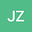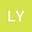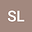A variable-order fractional $p(\cdot)$-Kirchhoff type problem in $\mathbb{R}^{N}$
•••Libo Yang
Hohai University
Author ProfileSihua Liang
Changchun Normal University
Author Profile## Abstract

This paper is concerned with the existence and multiplicity of solutions for the following variable $s(\cdot)$-order fractional $p(\cdot)$-Kirchhoff type problem \begin{equation*} \left\{\begin{array}{ll} M\left(\displaystyle\iint_{\mathbb R^{2N}}\frac{1}{p(x,y)}\displaystyle{\frac{|v(x)-v(y)|^{p(x,y)}}{|x-y|^{N+p(x,y)s(x,y)}}}dxdy\right)(-\Delta)^{s(\cdot)}_{p(\cdot)}v(x)+|v(x)|^{\overline{p}(x)-2}v(x) =\mu g(x,v)\ \ {\rm in}~\mathbb{R}^{N},\\ v\in W^{s(\cdot),p(\cdot)}(\mathbb{R}^{N}), \end{array}\right. \end{equation*} where $N>p(x,y)s(x,y)$ for any $(x,y)\in\mathbb{R}^{N}\times\mathbb{R}^{N}$, $(-\Delta)^{s(\cdot)}_{p(\cdot)}$ is a variable $s(\cdot)$-order $p(\cdot)$-fractional Laplace operator with $s(\cdot):\mathbb R^{2N}\to(0,1)$ and $p(\cdot):\mathbb R^{2N}\to(1,\infty)$, $\overline{p}(x)=p(x,x)$ for $x\in\mathbb{R}^{N}$, and $M$ is a continuous Kirchhoff-type function, $g(x,v)$ is a Carath\’{e}odory function, $\mu>0$ is a parameter. We obtain that there are at least two distinct solutions for the above problem by applying the generalized abstract critical point theorem. Under the weaker conditions, we also show the existence of one solution and infinitely many solutions by using the mountain pass lemma and fountain theorem, respectively. In particular, the new compact embedding result of the space $W^{s(\cdot),p(\cdot)}(\mathbb{R}^{N})$ into $L^{q(\cdot)}_{a(x)}(\mathbb{R}^{N})$ will be used to overcome the lack of compactness in $\mathbb{R}^N$. The main feature and difficulty of this paper is the presence of a double non-local term involving two variable parameters.
25 Jun 2020Submitted to Mathematical Methods in the Applied Sciences
27 Jun 2020Submission Checks Completed
27 Jun 2020Assigned to Editor
29 Jun 2020Reviewer(s) Assigned
02 Sep 2020Review(s) Completed, Editorial Evaluation Pending
02 Sep 2020Editorial Decision: Revise Minor